Next: SLA_PLANEL - Planet Position from Elements
Up: SUBPROGRAM SPECIFICATIONS
Previous: SLA_PERTEL - Perturbed Orbital Elements

## SLA_PERTUE - Perturbed Universal Elements

ACTION:
Update the universal elements of an asteroid or comet by applying planetary perturbations.

CALL:
CALL sla_PERTUE (DATE, U, JSTAT)

GIVEN:

 D DATE1 final epoch (TT MJD) for the updated elements

GIVEN and RETURNED:

 D(13) U universal elements (updated in place) (1) combined mass (M+m) (2) total energy of the orbit (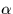) (3) reference (osculating) epoch (t0) (4-6) position at reference epoch (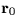) (7-9) velocity at reference epoch (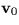) (10) heliocentric distance at reference epoch (11)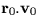(12) date (t) (13) universal eccentric anomaly (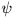) of date, approx

RETURNED:

 I JSTAT status: +102 = warning, distant epoch +101 = warning, large timespan (>100 years) +1 to +8 = coincident with major planet (Note 5) 0 = OK -1 = numerical error

NOTES:
1.
The universal'' elements are those which define the orbit for the purposes of the method of universal variables (see reference 2). They consist of the combined mass of the two bodies, an epoch, and the position and velocity vectors (arbitrary reference frame) at that epoch. The parameter set used here includes also various quantities that can, in fact, be derived from the other information. This approach is taken to avoiding unnecessary computation and loss of accuracy. The supplementary quantities are (i), which is proportional to the total energy of the orbit, (ii) the heliocentric distance at epoch, (iii) the outwards component of the velocity at the given epoch, (iv) an estimate of, the universal eccentric anomaly'' at a given date and (v) that date.
2.
The universal elements are with respect to the J2000 equator and equinox.
3.
The epochs DATE, U(3) and U(12) are all Modified Julian Dates (JD-2400000.5).
4.
The algorithm is a simplified form of Encke's method. It takes as a basis the unperturbed motion of the body, and numerically integrates the perturbing accelerations from the major planets. The expression used is essentially Sterne's 6.7-2 (reference 1). Everhart and Pitkin (reference 2) suggest rectifying the orbit at each integration step by propagating the new perturbed position and velocity as the new universal variables. In the present routine the orbit is rectified less frequently than this, in order to gain a slight speed advantage. However, the rectification is done directly in terms of position and velocity, as suggested by Everhart and Pitkin, bypassing the use of conventional orbital elements.

The f(q) part of the full Encke method is not used. The purpose of this part is to avoid subtracting two nearly equal quantities when calculating the indirect member'', which takes account of the small change in the Sun's attraction due to the slightly displaced position of the perturbed body. A simpler, direct calculation in double precision proves to be faster and not significantly less accurate.

Apart from employing a variable timestep, and occasionally rectifying the orbit'' to keep the indirect member small, the integration is done in a fairly straightforward way. The acceleration estimated for the middle of the timestep is assumed to apply throughout that timestep; it is also used in the extrapolation of the perturbations to the middle of the next timestep, to predict the new disturbed position. There is no iteration within a timestep.

Measures are taken to reach a compromise between execution time and accuracy. The starting-point is the goal of achieving arcsecond accuracy for ordinary minor planets over a ten-year timespan. This goal dictates how large the timesteps can be, which in turn dictates how frequently the unperturbed motion has to be recalculated from the osculating elements.

Within predetermined limits, the timestep for the numerical integration is varied in length in inverse proportion to the magnitude of the net acceleration on the body from the major planets.

The numerical integration requires estimates of the major-planet motions. Approximate positions for the major planets (Pluto alone is omitted) are obtained from the routine sla_PLANET. Two levels of interpolation are used, to enhance speed without significantly degrading accuracy. At a low frequency, the routine sla_PLANET is called to generate updated position+velocity state vectors''. The only task remaining to be carried out at the full frequency (i.e. at each integration step) is to use the state vectors to extrapolate the planetary positions. In place of a strictly linear extrapolation, some allowance is made for the curvature of the orbit by scaling back the radius vector as the linear extrapolation goes off at a tangent.

Various other approximations are made. For example, perturbations by Pluto and the minor planets are neglected, relativistic effects are not taken into account and the Earth-Moon system is treated as a single body.

In the interests of simplicity, the background calculations for the major planets are carried out en masse. The mean elements and state vectors for all the planets are refreshed at the same time, without regard for orbit curvature, mass or proximity.

5.
This routine is not intended to be used for major planets. However, if major-planet elements are supplied, sensible results will, in fact, be produced. This happens because the routine checks the separation between the body and each of the planets and interprets a suspiciously small value (0.001 AU) as an attempt to apply the routine to the planet concerned. If this condition is detected, the contribution from that planet is ignored, and the status is set to the planet number (Mercury=1,...,Neptune=8) as a warning.

REFERENCES:
1.
Sterne, Theodore E., An Introduction to Celestial Mechanics, Interscience Publishers, 1960. Section 6.7, p199.
2.
Everhart, E. & Pitkin, E.T., Am. J. Phys. 51, 712, 1983.Next: SLA_PLANEL - Planet Position from Elements
Up: SUBPROGRAM SPECIFICATIONS
Previous: SLA_PERTEL - Perturbed Orbital Elements

SLALIB --- Positional Astronomy Library